0

# 使用 Apache Spark 3.0 分析Stack Overflow数据集的保姆级教程

1. 从 Stack Exchange 存档下载数据转储（它是一个 7z 压缩的 XML 文件）
2. 解压下载的文件
3. 将文件上传到 S3（AWS 上的分布式对象存储）
4. 将 XML 文件转换为 Apache Parquet 格式（再次将 Parquet 保存在 S3 上）
5. 分析数据集

## 数据集

Stack Overflow 在程序员中非常流行，你可以在那里提问，也可以回答其他用户的问题。这个数据集本身可以在 Stack Exchange 存档中找到，并且在知识共享许可 cc-by-sa 4.0 下可用。

## 数据下载和解压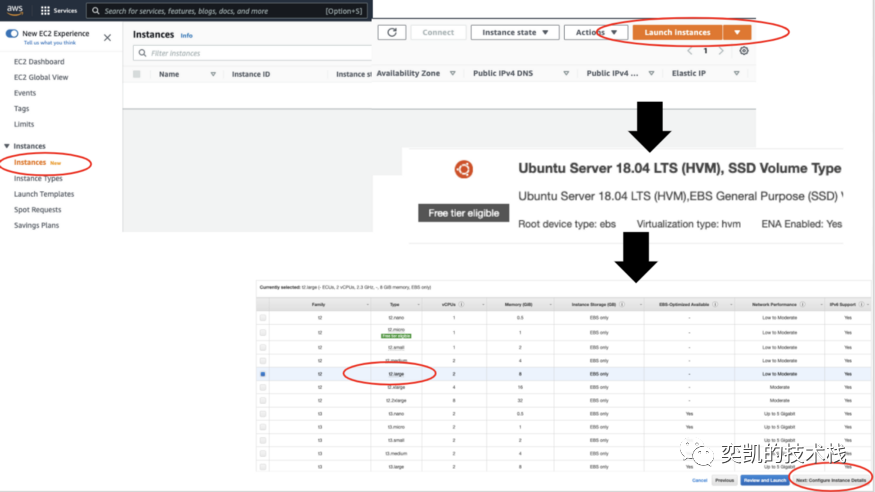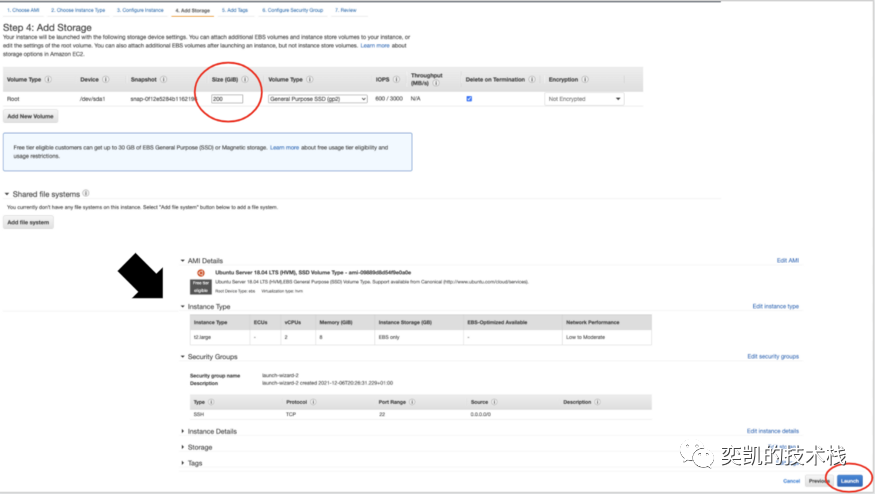AWS 会建议你设置一些安全组来限制可以使用实例的 IP 地址，因此请查看 AWS 文档。要启动实例需要有一个 EC2 密钥对，如果还没有可以生成它。 实例运行后，可以使用密钥从终端 ssh 链接到EC2实例。

``````[email protected]<the ip address of your instance>
``````

``````sudo apt update
sudo apt install python3-pip
sudo apt install p7zip-full
``````

``````pip3 install boto3
pip3 install requests
``````

``````from boto3.session import *
import os
access_key = '...'
secret_key = '...'
bucket = '...'
s3_output_prefix = '...'
session = Session(
aws_access_key_id=access_key,
aws_secret_access_key=secret_key
)
s3s = session.resource('s3').Bucket(bucket)

local_input_prefix = '/home/ubuntu'
file_name = 'Posts.xml'
input_path = os.path.join(local_input_prefix, file_name)
output_path = os.path.join(s3_output_prefix, file_name)
``````

## 数据预处理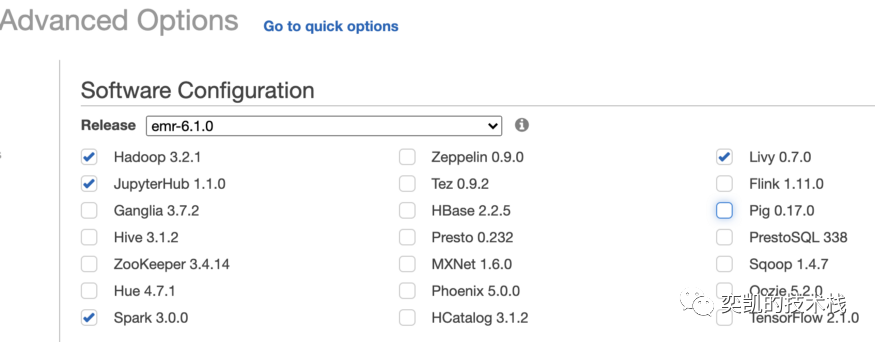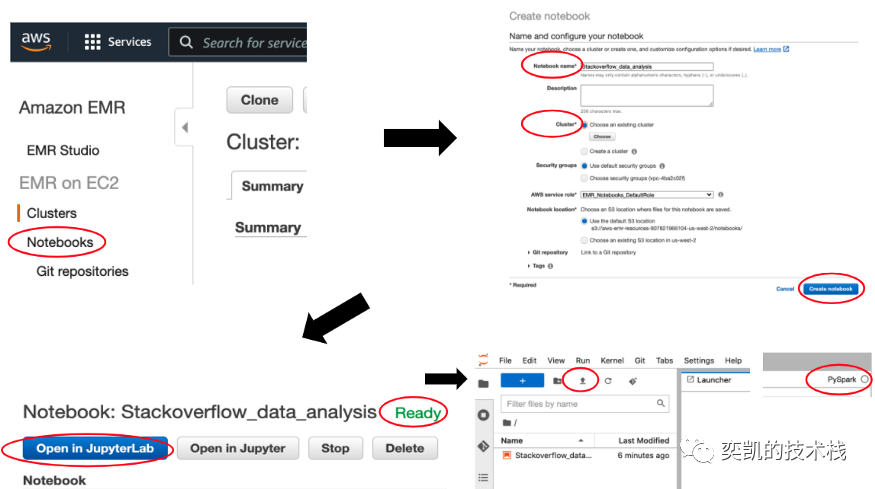``````Stackoverflow-Data-Analysis/data-prepare/data-prepare.ipynbStackoverflow-Data-Analysis/data-analysis/Posts-General-Analysis.ipynb
``````

``````posts_input_path = 's3://...'
posts_output_path = 's3://...'

(
spark
.format('xml')
.option('samplingRatio', 0.01)
.option('rowTag', 'row')
.select(
col('_Id').alias('id'),
(col('_CreationDate').cast('timestamp')).alias('creation_date'),
col('_Title').alias('title'),
col('_Body').alias('body'),
col('_FavoriteCount').alias('favorite_count'),
col('_OwnerDisplayName').alias('owner_display_name'),
col('_OwnerUserId').alias('user_id'),
col('_ParentId').alias('parent_id'),
col('_PostTypeId').alias('post_type_id'),
col('_Score').alias('score'),
col('_Tags').alias('tags'),
col('_ViewCount').alias('views')
)
.write
.mode('overwrite')
.format('parquet')
.option('path', posts_output_path)
.save()
)
``````

## 数据分析

1、计算计数

• 有多少问题
• 有多少答案
• 有多少问题已接受答案
• 有多少用户提出或回答了一个问题
``````posts_path = 's3://...'

posts = posts_all.select(
'id',
'post_type_id',
'user_id',
'creation_date',
'tags'
).cache()

posts.count()

questions = posts.filter(col('post_type_id') == 1)

questions.count()
posts.filter(col('user_id').isNotNull()).select('user_id').distinct().count()
``````

2、计算响应时间

``````response_time = (
questions.alias('questions')
.select(
col('questions.id'),
col('questions.creation_date').alias('question_time'),
)
.filter(col('response_time') > 0)
.orderBy('response_time')
)

response_time.show(truncate=False)
``````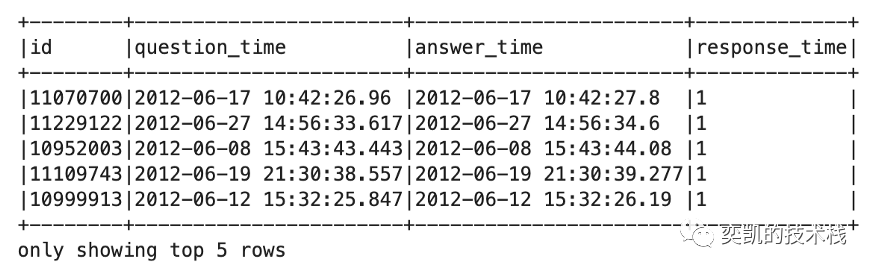``````hourly_data = (
response_time
.withColumn('hours', ceil(col('response_time') / 3600))
.groupBy('hours')
.agg(count('*').alias('cnt'))
.orderBy('hours')
.limit(24)
).toPandas()

hourly_data.plot(
x='hours', y='cnt', figsize=(12, 6),
title='Response time of questions',
legend=False,
kind='bar',
xlabel='Hour',
)
``````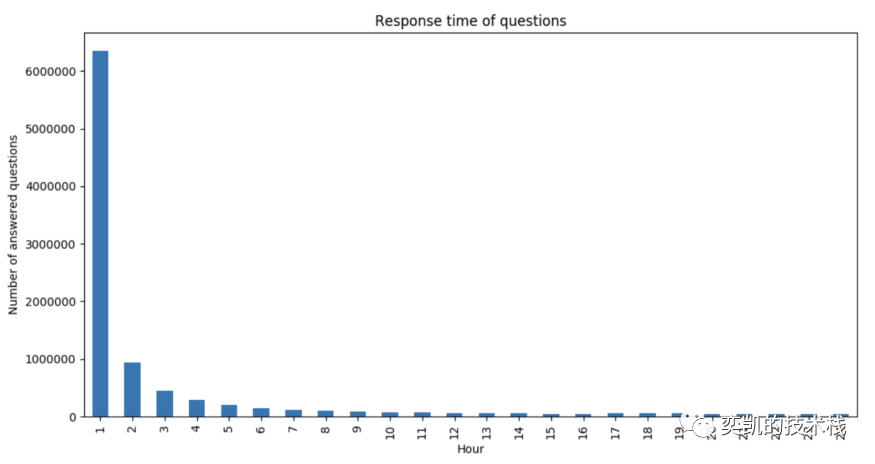3、查看问题/答案的数量如何随时间变化

``````posts_grouped = (
posts
.filter(col('user_id').isNotNull())
.groupBy(
window('creation_date', '1 week')
)
.agg(
sum(when(col('post_type_id') == 1, lit(1)).otherwise(lit(0))).alias('questions'),
)
.withColumn('date', col('window.start').cast('date'))
.orderBy('date')
).toPandas()

posts_grouped.plot(
x='date',
figsize=(12, 6),
legend=True,
xlabel='Date',
kind='line'
)
``````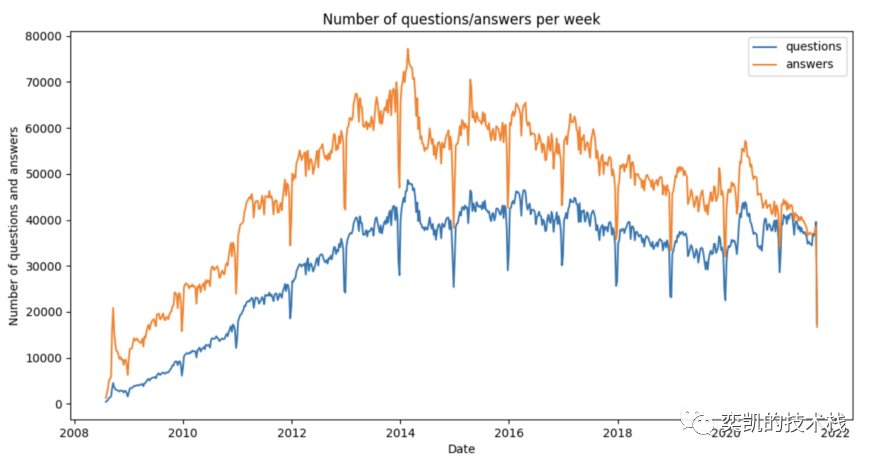4、计算标签的数量

``````tags = (
questions
.withColumn('tags', split('tags', '><'))
.selectExpr(
'*',
"TRANSFORM(tags, value -> regexp_replace(value, '(>|<)', '')) AS tags_arr"
)
)

tags.withColumn('tag', explode('tags_arr')).select('tag').distinct().count()

(
tags
.withColumn('tag', explode('tags_arr'))
.groupBy('tag')
.agg(count('*').alias('tag_frequency'))
.orderBy(desc('tag_frequency'))
).show(n=10)
``````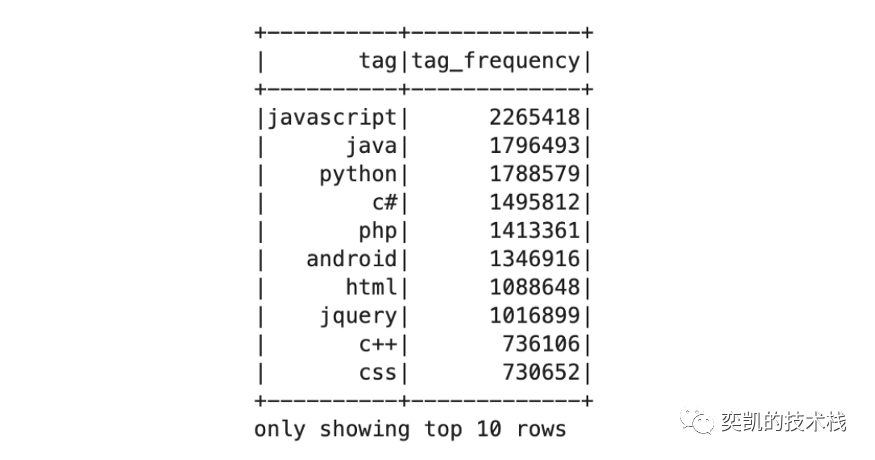5、查看一些特定标签的统计

``````spark_tag = (
tags
.filter(array_contains(col('tags_arr'), 'apache-spark') | array_contains(col('tags_arr'), 'apache-spark-sql'))
.groupBy(
window('creation_date', "1 week")
)
.agg(
count('*').alias('tag_frequency')
)
.withColumn('date', col('window.start').cast('date'))
.orderBy('date')
).toPandas()

spark_tag.plot(
x='date',
figsize=(12, 6),
title='spark/spark-sql tag frequency per week',
legend=False,
xlabel='Date',
ylabel='Number of questions with spark tag',
kind='line'
)
``````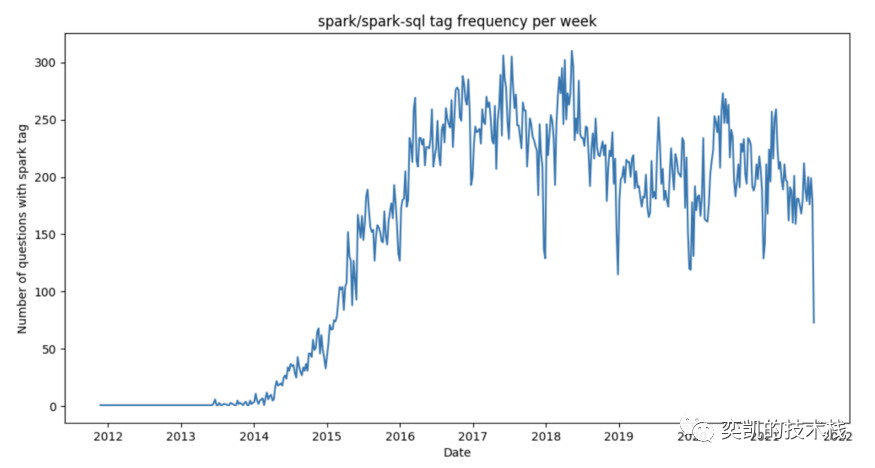## 总结

Stack Overflow 数据集承载了很多有趣的信息，我说的不仅仅是答案形式的内容，还包括问题、答案、评论和用户之间的一些有趣的关系。在本文中只使用 Spark 分析了数据集中的帖子数据，这只展示了冰山一角，在以后的一些帖子中，我们将应用一些更高级的分析工具，例如机器学习和 使用 Spark 进行图处理以发现数据的更多属性。

https://github.com/davidvrba/Stackoverflow-Data-Analysis

### “使用 Apache Spark 3.0 分析Stack Overflow数据集的保姆级教程”的评论:

##### 关于作者##### 奕凯